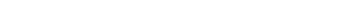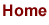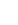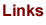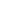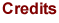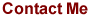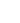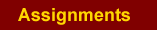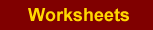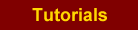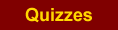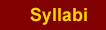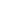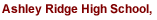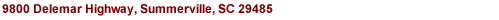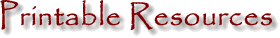The files on this page are .pdf files. You will need Adobe Acrobat. If you do not have Acrobat Reader version 6.0 or higher, click here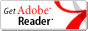and download the latest version of the completely free Acrobat Reader.
 [Graph Paper] [Algebra 1] [Algebra 2] [Geometry] [Pre-Calculus] [AP Calculus] [TI 83+] Graph Paper Unlabeled 15 - degree Polar Graph Paper 4 "7 x 7" Coordinate Axes 1/4 inch (whole sheet) 9 "5 x 5" Coordinate Axes Algebra 1 What to do with Signed Numbers?! Completing the Square Notes Algebra 2 Geometry Angle Puzzle Logic Lesson 1 Constructions Lesson 1 Logic Lesson 1 Assignment Constructions Lesson 2 Logic Lesson 2 Constructing A Parallel Line Venn diagram for quadrilaterals Hints for Proofs of Triangles Pre-Calculus AP Calculus Unit 1 Review Worksheet Unit 1 Notes Using the TI-83+ / TI-84+ Solve Systems of Linear Equations with Matrices Finding the Linear Regression Line